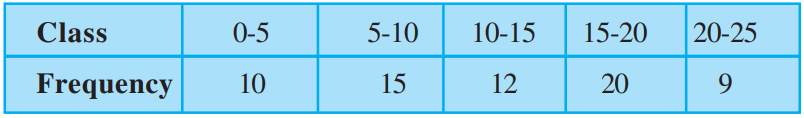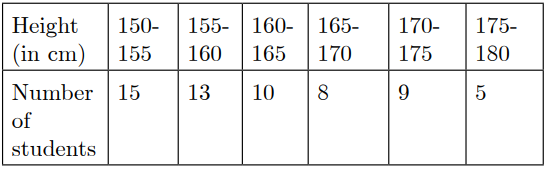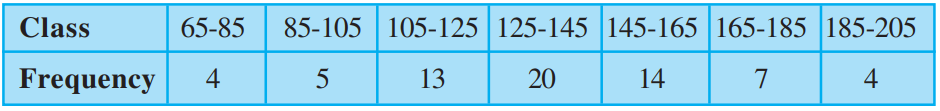Friday, October 22, 2021
Home > CBSE Class 10 > MCQ Questions for Class 10 Maths Chapter 14 Statistics with Answer Keys

# MCQ Questions for Class 10 Maths Chapter 14 Statistics with Answer KeysHi students, Welcome to AMBiPi (Amans Maths Blogs). In this article, you will get MCQ Questions for Class 10 Maths Chapter 14 Statistics with Answer Keys PDF. You can download this PDF and save it in your mobile device or laptop etc.

MCQ Questions for Class 10 Maths Chapter 14 Statistics Question No 1:

The median and mode respectively of a frequency distribution are 26 and 29, Then its mean is

Option A : 27.5

Option B : 24.5

Option C : 28.4

Option D : 25.8

Option B : 24.5

MCQ Questions for Class 10 Maths Chapter 14 Statistics Question No 2:

The abscissa of the point of intersection of the less than type and of the more than type cumulative frequency curves of a grouped data gives its

Option A : Mean

Option B : Median

Option C : Mode

Option D : All of Above

Option B : Median

MCQ Questions for Class 10 Maths Chapter 14 Statistics Question No 3:

One of the methods for determining mode is

Option A : Mode = 2 Median – 3 Mean

Option B : Mode = 3 Median – 2 Mean

Option C : Mode = 2 Mean – 3 Median

Option D : Mode = 3 Mean – 2 Median

Option B : Mode = 3 Median – 2 Mean

MCQ Questions for Class 10 Maths Chapter 14 Statistics Question No 4:

In a frequency distribution, the mid value of a class is 10 and the width of the class is 6. The lower limit of the class is

Option A : 6

Option B : 7

Option C : 8

Option D : 12

Option B : 7

MCQ Questions for Class 10 Maths Chapter 14 Statistics Question No 5:

For the following distribution :the sum of lower limits of the median class and modal class is

Option A : 15

Option B : 25

Option C : 30

Option D : 35

Option B : 25

MCQ Questions for Class 10 Maths Chapter 14 Statistics Question No 6:

The algebraic sum of the deviations of a frequency distribution from its mean is always,

Option A : greater than zero

Option B : less than zero

Option C : zero

Option D : a non-zero number

Option C : zero

MCQ Questions for Class 10 Maths Chapter 14 Statistics Question No 7:

Consider the following frequency distribution of the heights of 60 students of a class.The upper limit of the median class in the given data is

Option A : 165

Option B : 155

Option C : 150

Option D : 170

Option A : 165

MCQ Questions for Class 10 Maths Chapter 14 Statistics Question No 8:

Consider the following frequency distribution :The difference of the upper limit of the median class and the lower limit of the modal class is

Option A : 0

Option B : 19

Option C : 20

Option D : 38

Option C : 20

MCQ Questions for Class 10 Maths Chapter 14 Statistics Question No 9:

Which of the following can not be determined graphically?

Option A : Mean

Option B : Median

Option C : Mode

Option D : None of Above

Option A : Mean

MCQ Questions for Class 10 Maths Chapter 14 Statistics Question No 10:

If the difference of mode and median of a data is 24, then the difference of median and mean is

Option A : 12

Option B : 24

Option C : 8

Option D : 36